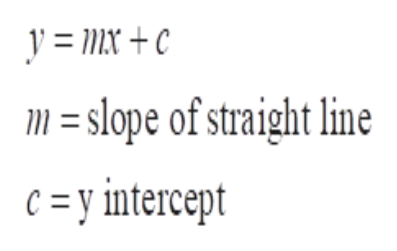# Write a function that models the distance D from a point on the line y = 9 x - 2 to the point (0,0) (as a function of x).D(x) =  The point on the line that is closest to (0, 0) is ( , ) (numerical answers)

Question
221 views

Write a function that models the distance D from a point on the line y = 9 x - 2 to the point (0,0) (as a function of x).

D(x) =

The point on the line that is closest to (0, 0) is

check_circle

Step 1

The general equation for the straight line is y = mx+c

Where m = slope of the straight line, c = y-intercepthelp_outlineImage Transcriptionclosey = Mx + c line m = slope of straight c =y intercept fullscreen
Step 2

Compare the general equation with the given equation

So,m = 9 and c = -2

Step 3

Since we need the model from distance D from a point from the line y = 9x – 2 and passing from the point (0,0).

Therefore, th...

### Want to see the full answer?

See Solution

#### Want to see this answer and more?

Solutions are written by subject experts who are available 24/7. Questions are typically answered within 1 hour.*

See Solution
*Response times may vary by subject and question.
Tagged in

### Calculus